# Kinematic characteristics

## Introduction

In case of magneto-mechanical coupling it is necessary to define the kinematic characteristics of the moving part and of the coupled load, in order to solve the dynamics equation.

## Handling in Flux

The diagram below shows the handling of motion in Flux:

• the fixed part, the moving part and the compressible area belong to the finite element domain
• the moving part is mechanically coupled to an external device that is the coupled load, outside the finite element domain## Mechanical and kinematic characteristics

The mechanical and kinematic characteristics necessary to solve the fundamental dynamics equation are the following:

• mass or moment of inertia of the moving part and of the coupled load
• resistant force or torque acting on the moving part and on the coupled load
• initial conditions: initial position and initial speed of the moving part

## Resistant force or torque

The resistant force or torque can be described in several ways, such as:

• predefined formulas: friction force, return force (spring)
• a varying formula, entered by the user

These two types of formulas are detailed in the following blocks.

## Predefined formulas (1)

The force or the resistive torque can be described by means of a predefined formula of friction force or torque type.

(1) Friction force or torque
Translation Rotation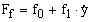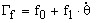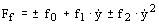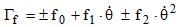The signs in the equation are defined in the following way:

• if speed is positive (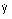or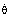> 0): the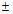sign is replaced by the + sign
• if speed is negative (or< 0): thesign is replaced by the – sign

The three coefficients f0, f1 and f2, are as follows:

• f0: constant friction coefficient, independent of speed (in N or N.m)

• f1: viscous friction coefficient, function of speed (in N.s/m or in N.m.s/degrees)

• f2: friction coefficient, function of speed to the second power (in N.s²/m² or in N.m.s²/degrees²)

Note: If there are symmetries and periodicities:

the coefficients f0, f1 and f2 must be defined for the entire device, even if it is not represented entirely. The forces or torques are computed for the entire device.

## Predefined formulas (2)

The force or the resistive torque can be described by means of a predefined formula of return force or torque type.

(2) Return force or torque (spring)
Translation Rotation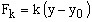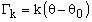The coefficient k is the stiffness (or torsion) constant of the spring

(in N/m or N.m/degree).

## Formula with I/O parameters

The force or the resistive torque can be described by the intermediary of a formula with I/O parameters.

A formula with I/O parameters is a formula function of kinematic parameters, such as position, speed, acceleration of the mechanical set considered; or a function of other mechanical sets; or a function of any parameters.